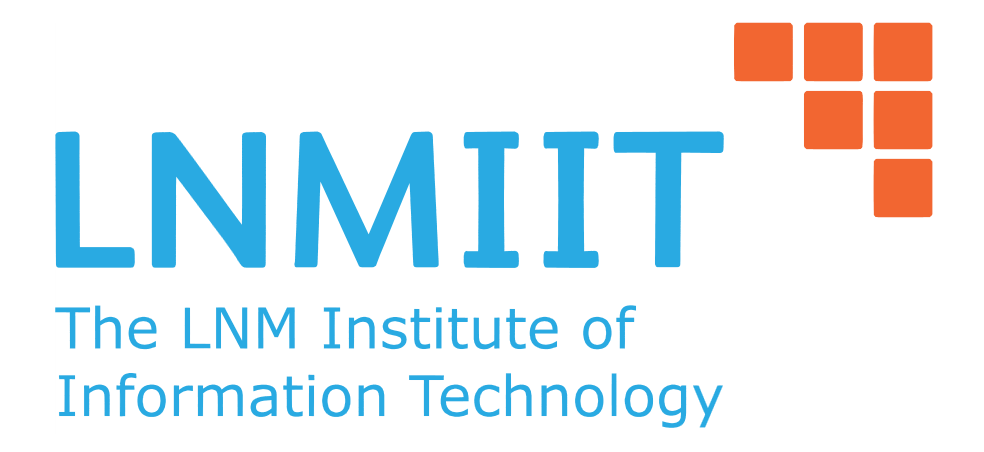Inversion Number
Tag(s):

## Easy, Number Theory, easy-medium, inclusion-exclusion

Problem
Editorial
Analytics

Zoro while studying numbers, observed an interesting property. He found out that every number can be represented as powers of primes. Now getting bored from studying, he thought of having some fun and defined an Inversion Number.

An Inversion Number of a number is defined as interchanging the power of the prime with the prime number in the prime factorization of the number.

Eg: $24= 2^{3}$ x $3^{1}$

Inversion Number of $(24)= 3^{2}$ x $1^{3}$ 9

Zoro likes a number who's inversion number is unity. He calls them Perfect Inversion Numbers.
Now Zoro wonders given a range L to R what are the number of perfect inversion numbers in that range.

Note

Inversion number of 0 is 0 and inversion number of 1 is 1.

Input

First line contains an integer T denoting the number of testcases. Next T lines contains 2 integers each the range L and R.

Output

The number of Perfect Inversion Number between the the range L and R (both inclusive).

Constraints:

$1\leq T \leq 10^{6}$
$0\leq L\leq R\leq 10^{6}$

NOTE Use Fast I/O

SAMPLE INPUT
2
1 3
4 5
SAMPLE OUTPUT
3
1
Explanation

In the first case, $1= 1^{1}$ , $2= 2^{1}$, $3=3^{1}$ Therefor the inversion number of all the 3 is 1. Therefore all the 3 have perfect inversion number.

Second case, Only 5 has a perfect inversion number. Therefore answer is 1.

Time Limit: 1,2 sec(s) for each input file.
Memory Limit: 256 MB
Source Limit: 1024 KB
Marking Scheme: Marks are awarded when all the testcases pass.
Allowed Languages: Bash, C, C++, C++14, Clojure, C#, D, Erlang, F#, Go, Groovy, Haskell, Java, Java 8, JavaScript(Rhino), JavaScript(Node.js), Julia, Kotlin, Lisp, Lisp (SBCL), Lua, Objective-C, OCaml, Octave, Pascal, Perl, PHP, Python, Python 3, R(RScript), Racket, Ruby, Rust, Scala, Swift, Swift-4.1, TypeScript, Visual Basic

## CODE EDITOR

Initializing Code Editor...

## This Problem was Asked inChallenge Name

LNMIIT Algorithms Cup - June 2017

OTHER PROBLEMS OF THIS CHALLENGE
• Algorithms > Graphs
• Math > Number Theory
• Data Structures > Advanced Data Structures
• Algorithms > Graphs
Уведомления

?